Next: Boundary Value Problems Up: Electrostatic Fields Previous: Charge Sheets and Dipole

# Green's Theorem

Consider the vector identity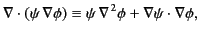(216)

where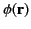and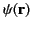are two arbitrary (but differentiable) vector fields. We can also write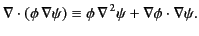(217)

Forming the difference between the previous two equation, we get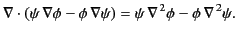(218)

Finally, integrating this expression over some volume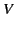, bounded by the closed surface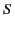, and making use of the divergence theorem, we obtain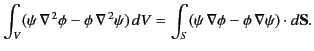(219)

This result is known as Green's theorem.

Changing the variable of integration, the above result can be rewritten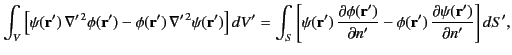(220)

where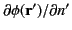is shorthand for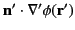, et cetera. Suppose thatis a solution to Poisson's equation,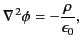(221)

associated with some charge distribution,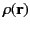, that extends over all space, subject to the boundary condition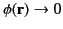as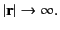(222)

Suppose, further, that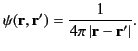(223)

It follows from Equation (25), and symmetry, that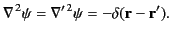(224)

The previous five equations can be combined to give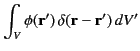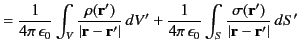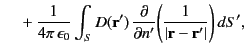(225)

where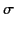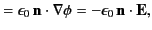(226)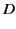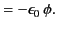(227)

It follows, by comparison with Equations (162), (202), and (205), that the three terms on the right-hand side of Equation (225) are the electric potential generated by the charges distributed within, the potential generated by the surface charge distribution,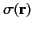, on, and the potential generated by the surface dipole distribution,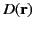, on, respectively.

Suppose that the point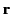lies within. In this case, Equation (225) yields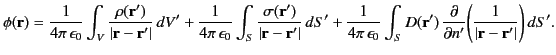(228)

However, we know that the general solution to Equation (221), subject to the boundary condition (222), is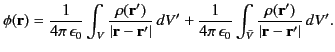(229)

Here,denotes the region of space lying within the closed surface, whereas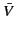denotes the region lying outside. A comparison of the previous two equations reveals that, for a pointlying within,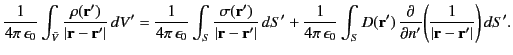(230)

In other words, the electric potential (and, hence, the electric field) generated withinby the charges external tois equivalent to that generated by the charge sheet, and the dipole sheet, distributed on. Furthermore, becausedepends on the normal derivative of the potential at, whereas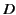depends on the potential at, it follows that we can completely determine the potential withinonce we known the distribution of charges within, and the values of the potential and its normal derivative on. In fact, this is an overstatement, because the potential and its normal derivative are not independent of one another, but are related via Poisson's equation. In other words, a knowledge of the potential onalso implies a knowledge of its normal derivative, and vice versa. Hence, we can, actually, determine the potential withinfrom a knowledge of the distribution of charges inside, and the distribution of either the potential, or its normal derivative, on. The specification of the potential onis known as a Dirichlet boundary condition. On the other hand, the specification of the normal derivative of the potential onis called a Neumann boundary condition.

Suppose that the pointlies outside. In this case, Equation (225) yields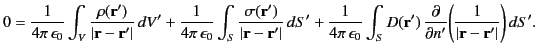(231)

In other words, outside, the electric potential generated by the surface charge distribution, combined with that generated by the surface dipole distribution, completely cancels out the electric potential (and, hence, the electric field) produced by the charges distributed within. As an example of this type of cancellation, suppose thatis a spherical surface of radius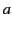, centered on the origin. Within, let there be a single charge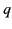, located at the origin. The electric field and potential generated by this charge are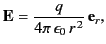(232)

and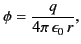(233)

respectively. It follows from Equations (226) and (227) that the densities of the charge and dipole sheets at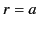that are needed to cancel out the effect of the central charge in the region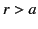are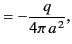(234)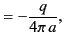(235)

respectively. Making use of Equations (212)-(215), and (232)-(235), the electric field and potential generated by the combination of the charge at the origin, the charge sheet at, and the dipole sheet at, are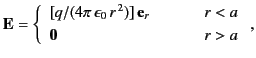(236)

and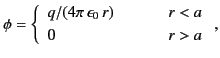(237)

respectively. We can see that the charge and dipole sheet atdo not affect the electric field, or the potential, due to the central charge in the region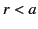, but completely cancel out this charge's field and potential in the region.Next: Boundary Value Problems Up: Electrostatic Fields Previous: Charge Sheets and Dipole
Richard Fitzpatrick 2014-06-27